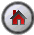SHAZAM Time-Series Cross-Section Data

## Pooling Time-Series Cross-Section Data

Data sets may combine time series and cross section data. Two types of data sets are:

1. A data set with cross-sections such as states, provinces or countries.
2. A micro-panel or longitudinal data set constructed from a survey of the same micro-units over time. This type of data may typically contain a large number of cross-sectional units (for example, families or individuals) and relatively few time periods.

A pooled regression model assumes common coefficients across the cross-section units. The `POOL` command in SHAZAM provides features for estimating models that combine time series and cross section data. The general command format is:

 `POOL depvar indeps / NCROSS=n options `

where depvar is the dependent variable, indeps is a list of the explanatory variables and options is a list of desired options. The `NCROSS=` option specifies the number of cross-section units. The data must be arranged so that all observations of a cross-section are together. That is, the complete time series for the first group must be followed by the complete time series for the second group etc.

#### Examples

The examples in this section use the investment demand data set that is analyzed in Greene [2000, Chapter 15].

#### References

Nathaniel Beck and Jonathan N. Katz, "What to do (and not to do) with Time-Series Cross-Section Data", American Political Science Review, Vol. 89, 1995, pp. 634-47.

Nathaniel Beck, Jonathan N. Katz, R. Michael Alvarez, Geoffrey Garrett and Peter Lange, "Government Partisanship, Labor Organization and Macroeconomic Performance: A Corrigendum", American Political Science Review, Vol. 87, 1993, pp. 945-948.

A. Bhargava, L. Franzini and W. Narendranathan, "Serial Correlation and the Fixed Effects Model", Review of Economic Studies, Vol. 49, 1982, pp. 533-549.

A. Buse, "Goodness of Fit in Generalized Least Squares Estimation", American Statistician, Vol. 27, 1973, pp. 106-108.

William H. Greene, Econometric Analysis, Fourth Edition, 2000, Prentice-Hall.

J. Kmenta, Elements of Econometrics, 1986, Macmillan.

Richard W. Parks, "Efficient Estimation of a System of Regression Equations when Disturbances are both Serially and Contemporaneously Correlated", Journal of the American Statistical Association, Vol. 62, 1967, pp. 500-509.[SHAZAM Guide home]

### Including lagged variables as explanatory variables

When the `POOL` command is used, if lagged variables are included as explanatory variables then they should be specified using the special form:

```         var(first.last)
```

where `var` is a variable name and the numbers in parentheses specify the `first` and `last` periods to use for lags. For example, the next command implements pooled estimation with a lagged dependent variable.

 ```POOL Y Y(1.1) X / NCROSS=4 ```

### Estimation with a sub-set of time series observations

When the `POOL` command is used, a sub-set of time series observations can be selected. This is demonstrated in the next list of SHAZAM commands.

 ```SAMPLE 1 100 * Set the number of time periods GEN1 NT=20 * Generate an index for each cross-section GENR CSINDEX=SUM(SEAS(NT)) * Generate a time index for each cross-section GENR TINDEX=TIME(0)-NT*(CSINDEX-1) * Estimate over the time period 1 to 15 SET NOWARNSKIP SKIPIF (TINDEX.GT.15) POOL I F C / NCROSS=5 DELETE SKIP\$ STOP ```